# How RC4 Works

Now that you understand the very basics of encryption, let's take a closer look at how it is implemented in RC4. RC4 is a synchronous stream cipher that uses XOR to combine output from a key stream generator with the plaintext of the message. When used properly, its state value is relatively unpredictable; thus, it is a strong, fast, and valuable method for encrypting data. This algorithm was created by RSA Data Security, but was leaked on a newsgroup in 1994. Although the algorithm is a trade secret of RSA, RC4 has found its way into several technologies. These technologies include Secure Sockets Layer (SSL) and WEP.

The leaked RC4 algorithm is commonly referred to as ARC4 (assumed RC4). RSA never acknowledged that the leaked algorithm was RC4, but it has been shown to be functionally equivalent to RC4. Real RC4 requires licensing from RSA, while open -source based RC4 products use the leaked ARC4 algorithm.

There are several components to the RC4 algorithm. Each part plays an important role in the capability of RC4 to encrypt and decrypt data. The following will break each of these parts down so you can understand the process through which data must pass before it is fully encrypted.

#### RC4 Encryption States

RC4 uses a concept known as states in its encryption and decryption process. The state value is held in array, which is a matrix of values. For example, an alphabet array would hold the values "a “z". A particular letter could be called from the array by using the respective number of the alphabet. In other words, alpha(1) would = "a", and alpha(26) would = "z".

In addition, an array can be useful if a series of values needs to be swapped, or if you require some form of random value generation. For example, what if the values of the alpha array were randomized? This would provide the user a random alphabetical value if she called on alpha(1).

This type of random generation is used in RC4. During the encryption process, a series of numerical values (usually 1 “256) is placed into a state array, which is then scrambled. The state array is then used in the key stream, which is discussed later.

#### Initiation Vector Used in RC4

When data is passed through the RC4 algorithm, there are two pieces of information that go into the encryption process. The first is the password, which is required by both parties to encrypt and decrypt the data. However, because of the transmission method of data encrypted with RC4, it would be a simple matter to capture and crack ciphertext if only the password was used to encrypt the data. A hacker would simply have to determine the value of the plaintext prior to its encryption, then capture the transmitted ciphertext and compare the two. The resulting difference would easily yield the password.

What makes RC4 a streaming cipher is its use of a randomly changing value in the encryption process. This value, known as the initiation vector (IV) , is calculated using the previously discussed state array and the properties of the password, such as length and character value. Depending on the implementation of the RC4 algorithm, the IV could be a short byte- sized value, or a combination of many bytes (called words). In the case of WEP, the IV is 24 bits (3 bytes). The IV is either prefixed or post-fixed to the ciphertext and sent to the recipient, who will need the value to reverse the encryption process.

The IV is supposed to create a new key to avoid re-use of the secret key when the state table is re- initialized for each packet/block of inbound data. This creates a unique state table for each packet. Each packet makes the encryption process start at the beginning of the S array and then walk thru it. If the same secret key is used to build the S array, then both packets will use the exact same values for encrypting the input packets/blocks. This is why the shared key/small IV space is so dangerous, because the IV + secret key used to build the S array will repeat often.

The party who receives the encrypted text requires both the password and the IV to initialize the decryption process. As we previously mentioned, an internal state is used in the encryption process to create the streaming cipher. This means the same internal state must also be created on the decrypting side to allow reversal of the algorithm. Without this IV, the RC4 algorithm would not know which value in the array was used to create the cipher.

#### Key Scheduling Algorithm Generation in RC4

Now that you understand the purpose of the internal state and the purpose of the IV, let's take a closer look at the actual encryption process.

The first part of the encryption algorithm generates the Key Scheduling Algorithm (KSA) . This is accomplished by creating an array of values equal to the index you want to use in the algorithm. RC4 comes in several varieties: 8-bit, 16-bit, and so on. WEP uses 8-bit RC4 and operates on 8-bit values by creating an array with 256 8-bit values for a lookup table (8-bits of 8-bit values).

The next step of the KSA is to scramble the array. This is accomplished by using the password's character values in combination with a loop equal to the previously mentioned index. After several hundred addition and swap commands, the state array becomes thoroughly jumbled. Once this is complete, the algorithm moves to the actual encryption process (see Listing 4.1).

##### Listing 4.1 Key Scheduling Algorithm
` Initialization:  For i = 0 ... N - 1          S[i] = i        j = 0  Scrambling:        For i = 0 ... N - 1          j = j + S[i] + K[i mod l]         Swap(S[i], S[j]) `

#### Pseudo Random Generation Algorithm: Generating the Streaming Key

After the state array has been computed, it is time to move on to the encryption process. This part of the algorithm is responsible for creating the streaming values used to encrypt the plaintext, which is based on the output of the KSA. The stream is created by looping through the algorithm, provided in Listing 4.2, for each byte of the packet. This streaming value is then used in an XOR calculation against the plaintext. The result is ciphertext, which is sent to the receiving party.

##### Listing 4.2 Pseudo Random Generation Algorithm (PRGA)
` Initialization:  i = 0        j = 0  Generation Loop:        i = i + 1        j = (j + S[i]) mod l        Swap(S[i], S[j])        Output z = S[S[i] + S[j]] `

#### An Example

To illustrate how this works on a basic level, we are going to walk through the actual process of encrypting the word HI . In our example, the password is 6152 , which could be a birthday or someone's anniversary.

##### Creation of the State Array

To create the state array, several values must be known. These are the initial value of the variable i and j , the index value, and the password. In our example, we will assume both i and j are both , and the index value is 4 . We choose 4 because of the impossibility of attempting to manually illustrate RC4 using a normal index value of 256.

It would take far too long to work through all the KSA steps with normal 8-bit RC4. It is far easier to demonstrate the KSA process with a much smaller S array size value like 4, so we will assume that we are using 2-bit RC4 with this example.

The initial values of our variable are as follows :

` i=0    j=0    pass="6152"    pass length=4    Index (N) = 4 `

Now, let's initialize the KSA:

` For i = 0 ... N - 1                    S[i] = i  Next `

In regular English, this would read "Continue this loop until i=N-1 (or i=4-1 ), adding one to i each time through the loop and adding the current value of i to the state array ( S[i] )."

In other words, once this loop was complete, we would have the following values assigned to the state array:

` S=0    S=1    S=2    S=3 `

Next we need to scramble the values held in the state array using the following algorithm:

` For i = 0 ... N - 1                    j = j + S[i] + K[i mod l]               Swap(S[i], S[j]) `

In English, this says "Continue this loop until i=N-1 (or i=4-1 ), adding one to i each time through the loop. For every time through the loop, calculate the value of j , and then swap the array value held in S[i] for the value held in S[j] ."

#### KSA Example

To illustrate, we will present the current values of each variable prior to each pass through the loop, as well as the values after they have been processed in the loop.

Note: the term mod means to output the remaining value AFTER a division has occurred. In other words, 4 mod 4 would result in 0. The mod does not contribute to the encryption/scrambling function of the cipher, but instead keeps the calculation within the defined scope, which is set by the Index value (256). Without this mod process, the calculations would produce values that are greater than the size of our key array, resulting in program crashes and/or corruption.

In other words, if the results of a calculation were 6, and the modulus was 4, the resulting value would be 2 (6/4 = 1 with 2 remaining).

##### First Loop

Initial values:

` S=0   S=1    S=2    S=3  K=6    K=1    K=5    K=2  i=0    j=0    pass (K)="6152"    pass length(l)=4    Index (N) = 4 `

Equations:

` j = j + S[i] + K[i mod l]  Swap(S[i], S[j])  j=(0 + S + K) mod 4  j=(0+0+6) mod 4  j=6 mod 4  j=2  Swap (S , S)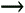S=0 , S=2S=2 , S=0 `

Final values:

` S=2    S=1    S=0    S=3  K=6    K=1    K=5    K=2  i=1    j=2    pass (K)="6152"        pass length(l)=4    Index (N) = 4 `
##### Second Loop

Initial values:

` S=2    S=1    S=0    S=3  K=6    K=1    K=5    K=2  i=1    j=2    pass (K)="6152"        pass length(l)=4    Index (N) = 4 `

Equations:

` j = j + S[i] + K[i mod l]  Swap(S[i], S[j])  j=(2 + S + K) mod 4  j=(2+1+1) mod 4  j=4 mod 4  j=0  Swap (S , S)S=1 , S=2S=2 , S=1 `

Final values:

` S=1    S=2    S=0    S=3  K=6    K=1    K=5    K=2  i=2    j=0    pass (K)="6152"    pass length(l)=4    Index (N) = 4 `
##### Third Loop

Initial values:

` S=1    S=2    S=0    S=3  K=6    K=1    K=5    K=2  i=2    j=0    pass (K)="6152"    pass length(l)=4    Index (N) = 4 `

Equations:

` j = j + S[i] + K[i mod l]  Swap(S[i], S[j])  j=(0 + S + K) mod 4  j=(0+0+5) mod 4  j=5 mod 4  j=1  Swap (S , S)S=0 , S=2S=2 , S=0 `

Final values:

` S=1    S=0    S=2    S=3  K=6    K=1    K=5    K=2  i=3    j=1    pass (K)="6152"    pass length(l)=4    Index (N) = 4 `
##### Fourth Loop

Initial values:

` S=1    S=0    S=2    S=3  K=6    K=1    K=5    K=2  i=3    j=1    pass (K)="6152"    pass length(l)=4    Index (N) = 4 `

Equations:

` j = j + S[i] + K[i mod l]  Swap(S[i], S[j])  j=(1 + S + K) mod 4  j=(1+3+2) mod 4  j=6 mod 4  j=2  Swap (S , S)S=3 , S=2S=2 , S=3 `

Final values:

` S=1    S=0    S=3    S=2  K=6    K=1    K=5    K=2  i=4    j=2    pass (K)="6152"    pass length(l)=4    Index (N) = 4 `

#### PRGA Example

Now that we have set up the KSA, it is time to initialize and use the PRGA. This uses the following algorithm, into which the values stored during the scheduling were placed.

Initialization:

` i = 0       j = 0 `

Generation Loop:

` i = i + 1  j = j + S[i]  Swap(S[i], S[j]) mod l  Output z = S[S[i] + S[j]] `
##### First Loop

Initial values:

` S=1    S=0    S=3    S=2  K=6    K=1    K=5    K=2  i=0    j=0    pass (K)="6152"    pass length(l)=4    Index (N) = 4 `

Algorithm:

` i=0+1=1  j=0+S=0+0=0  Swap (S , S)S=0 , S=1S=1 , S=0  z1=S[S+S]=S[0+1]=S=1  z1=00000001 `
##### Second Loop

Initial values:

` S=0    S=1    S=3    S=2  K=6    K=1    K=5    K=2  i=1    j=0    pass (K)="6152"    pass length(l)=4    Index (N) = 4 `

Algorithm:

` i=1+1=2  j=0+S=3  Swap(S,S)S=3, S=2S=2, S=3  z2=S[S+S] = S[3+2]=S%4=S=1  z2=00000001 `

Final Initial values:

` S=0    S=1    S=2    S=3  K=6    K=1    K=5    K=2  i=2    j=3    pass (K)="6152"    pass length(l)=4    Index (N) = 4 `

Algorithm:

` i=2+1=3  j=3+S=6%4=2  Swap(S,S)S=3, S=2S=2, S=3  z=S[S+S] = S[3+2]=S%4=S=1  1=00000001 `

And so on.

#### XOR PRGA with Plaintext Example

Now that we have a PRGA stream (albeit a basic one), let's use it to encrypt the word HI . Because RC4 is a bitwise encrypter, we need to convert the letters H and I to their binary values. The follow breaks down the conversion process:

` H (ASCII)072 (ANSI)1001000 (binary)  I (ASCII)073 (ANSI)1001001 (binary) `

Let's now XOR the binary values with the output stream from the PRGA.

` H (ASCII)072 (ANSI)01001000 (binary)                XOR     00000001 (value of z after first pass)                     01001001 (binary)072 (ANSI)I (ASCII)  I (ASCII)073 (ANSI)01001001 (binary)                XOR     00000001 (value of z after second pass)                     01001000 (binary)073 (ANSI)H (ASCII) `

Therefore, the encrypted value of HI when used with a simple form of RC4 is IH . Note that this is a very basic illustration that used an index of 4 and a short password. Typically, this index would be 256, and the password values would be anything from 1 “256. The password values are actually converted to their decimal equivalent of the ASCII characters entered by the end user.

#### RC4 and WEP

As previously mentioned, RC4 uses initialization vectors. This is a unique value that ensures each packet of information sent out is encrypted with a different key stream. Recalling our example, we used the password 6152 to encrypt the data. The values of each character and the length of the password were both used to set up the KSA, which in turn became the seed for the PRGA. The PRGA then created the streaming key data, which was XORed with the plaintext to produce the ciphertext. For the sake of keeping things as simple as possible, we only illustrated the encryption of one "packet" of data, using an unrealistic password.

In the real world, RC4 would be encrypting multiple packets. The IV is used to load the KSA with a different value for each and every different packet of information passed over the wireless network. This creates a different encryption stream (PRGA) for each chunk of data. The unique key stream is ultimately XORed with the plaintext, which finally produces the data sent out over the airwaves.

Although this creates a more secure environment for data transfer, it also requires the communicating parties to share two pieces of information. The first is the password, which is preshared and typically static. The second is the IV, which changes for each packet. This means the IV must also be sent out over the airwaves in such a way that the receiving party can determine its value.

So, what is the IV used for? Well, in our example, we used a 4-digit value as a password ( 6152 ). However, this is not a real-world example. In reality, a 5- or 13-digit password is used, which is then combined with the IV to create an 8- or 16-digit password. In other words, the IV becomes the part of the password that is used to generate the KSA, and ultimately the ciphertext.

#### Understanding Key Strength

RC4, as incorporated into WEP, uses either 40- or 104-bit protection with a 24-bit IV.

When you create a password, its letters and/or numbers are converted into their binary equivalent. To illustrate, we will convert the word HACKS into its binary equivalent.

` H(ASCII)072 (ANSI)01001000 (binary)  A(ASCII)065 (ANSI)01000001 (binary)  C(ASCII)067 (ANSI)01000011 (binary)  K(ASCII)075 (ANSI)01001011 (binary)  S(ASCII)083 (ANSI)01010011 (binary) `

Therefore,

` HACKS0100100001000001010000110100101101010011 `

Note that the binary equivalent of each letter contains eight bits. Also note, the entire password when converted to binary equals 40 bits (ones and zeros).

If you own a wireless device or have ever set up a wireless network, you will probably not recognize these 40- or 104-bit values. However, you might recognize the term 64-bit and 128-bit (Figure 4.2). These values are actually referring to the 40- and 104-bit encryption we just discussed. What the vendor is not telling you is that 24 bits of the encryption belong to the IVs prepended to the password during the encryption process. In other words, 64-bit encryption is created by a five letter-password and three IV values, and 128-bit encryption is created by a thirteen letter-password and three IV values. This is slightly misleading on the vendor's part, simply because the IV bits are not really secure. In fact, these values are sent over the airwaves in plain text!

##### Figure 4.2. Linksys WAP11 64-bit WEP key settings.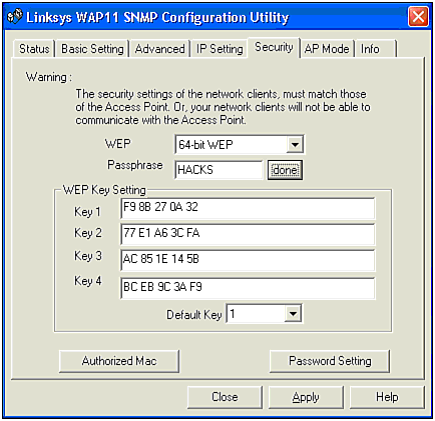NOTE

Not all implementations convert a ASCII string/pass phrase to a hex/binary key. Many APs require the user to enter hex key values for a full 40-bit or 104-bit key.

Many vendors have created their own processes by which they convert your chosen password into a 5- or 13-digit value, depending on the encryption strength. For example, if we enter the password HACKS into a Linksys WAP11 as the pass phrase, it hashes this value into four unique 40-bit keys, or four unique 104-bit keys, depending on the encryption strength. Although the Linksys configuration tool uses the same hash to configure client computers, other wireless card configuration tools might not. This means you are required to manually enter the 5- or 13-hex values created by the Linksys WAP11. If you note from the screenshots in Figure 4.2 and 4.3, Linksys is guilty of calling their encryption 64-bit. Although this is partially true, it is misleading to believe the strength is truly 64-bit. In fact, as we will learn later, even the 40-bit encryption used by many vendors is flawed, and actually reduces the generated keysize significantly. As a result of the reduced keysize, the encryption can often be nullified by brute-force key guessing.

##### Figure 4.3. Linksys WAP11 128-bit WEP key settings.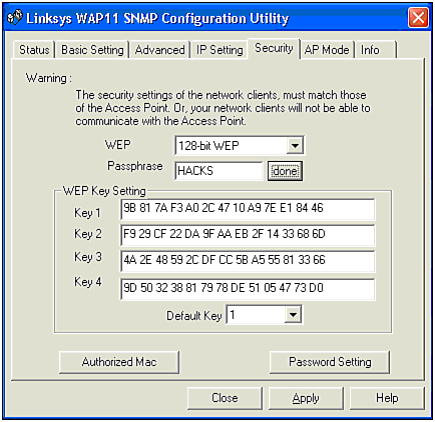#### Verifying Data Integrity Using Cyclic Redundancy Checks (CRC)

The first step in a wireless data transfer is to break the data into smaller chunks that can be transmitted. This is similar to the spoken language of humans . We speak in words, which the listener's brain reassembles into sentences and paragraphs. In the same way, TCP/IP-based wireless communication segments large chunks of data into smaller chunks .

When data is sent over the airwaves, or even through land-based wires, it must remain intact. For example, if you send an email containing a nuclear fission equation, and one crucial character of the equation is changed, the result could be chaotic . The same occurs when sending data. Thus the question arises, "How do we tell whether the data was corrupted in transfer?"

The answer is a checksum. The checksum is created using a simple algorithm that derives a unique number based on the specific data. In the case of 802.11, this value is a 32-bit (or 4-byte) value.

Once calculated, the checksum travels with the rest of the data transfer. This permits the receiving side to perform an integrity check. To do this, the recipient performs its own CRC-32 calculation and compares it with the original CRC-32 value. If the values match, the packet is intact.

#### The WEP Process

We have covered a great deal of information thus far in the chapter. You now understand how RC4 works, why the IV is important to the encryption process, and what role passwords play with WEP. You also understand that each packet not only goes through an encryption process, but also an integrity check. We will now put this entire process together by stepping through a live wireless data transmission.

First we need data. Suppose we send an Instant Message (IM) across a wireless network to ourself. This message is quite long, so our computer firsts breaks the data into several smaller and more manageable chunks of information, as illustrated in Figure 4.4. The data is then sent to the chat relay server, and sent back to us.

##### Figure 4.4. Data processing.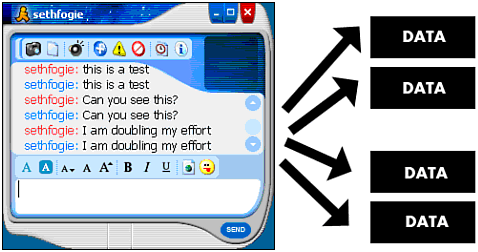As it processes the data, it takes the first chunk and passes it through the CRC-32 algorithm. This creates a value, which we will call CS (for checksum). The CS is then added onto the end of its corresponding data packet, as illustrated in Figure 4.5.

##### Figure 4.5. WEP checksumming .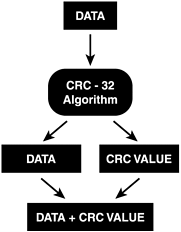After this is complete, the RC4 algorithm is called upon to encrypt the data. Using a changing series of initialization vectors in combination with the password shared between the two parties, the KSA creates the RC4 state values. These values then go into the PRGA, which creates the key stream. The key stream is then XORed with the DATA+CRC value to produce the ciphertext. Figure 4.6 illustrates this process.

##### Figure 4.6. WEP encryption.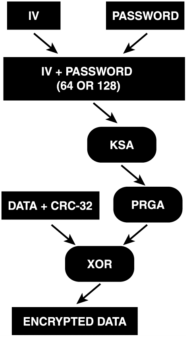We now have the encrypted data ready to send out over the airwaves. However, there is one final part that must be included with the data: the IV. Without this information, the receiving party would have no idea where to start the decryption process. Therefore, the three-byte IV is actually appended as plaintext to the encrypted data. This plaintext value is then used by the remote party to decrypt the frame, which is why it must be sent in the clear.

On the receiving side, this entire process is reversed . Fig. 4.7 illustrates the complex decryption process that occurs for each and every packet of data sent over a wireless network.

##### Figure 4.7. WEP decryption.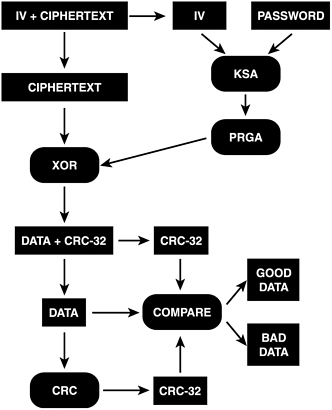To review, when a wireless device receives an encrypted packet, it first extracts the IV from the ciphertext and then combines it with the shared password. The resultant value is used to recreate the RC4 state used in the KSA, which in turn is used by the PRGA to create the key stream. The stream is then XORed with the ciphertext to create the plaintext, which contains the data and CRC-32 value of the data. The data is then separated and processed through the CRC algorithm again to create a new CRC-32 value, which is compared with the transmitted CRC-32 value. If the CRC-32 values match, the packet is considered valid; otherwise , the packet is dumped.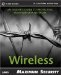Maximum Wireless Security
ISBN: 0672324881
EAN: 2147483647
Year: 2002
Pages: 171

Similar book on Amazon